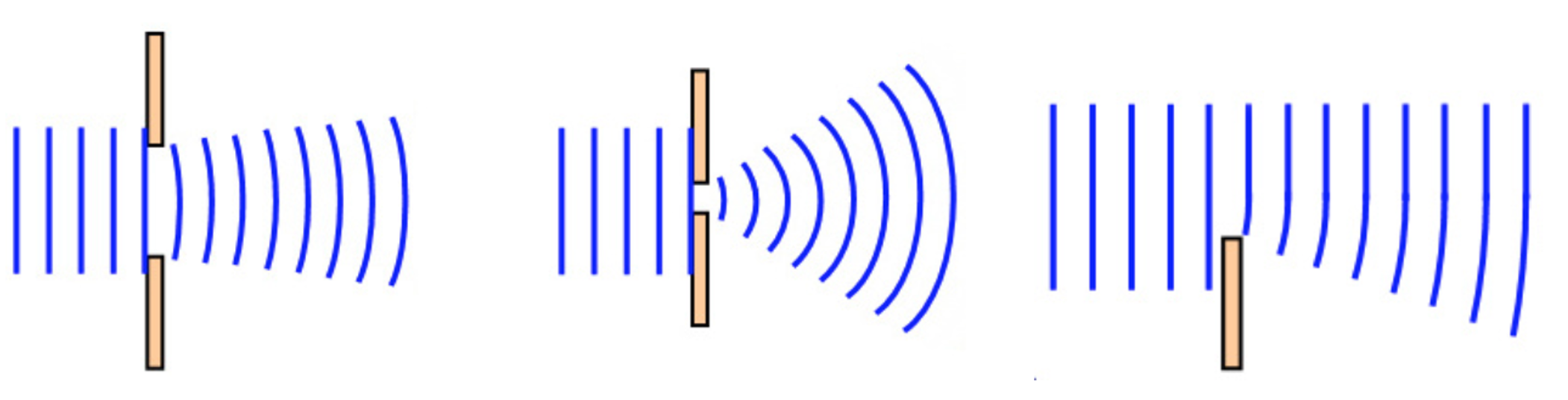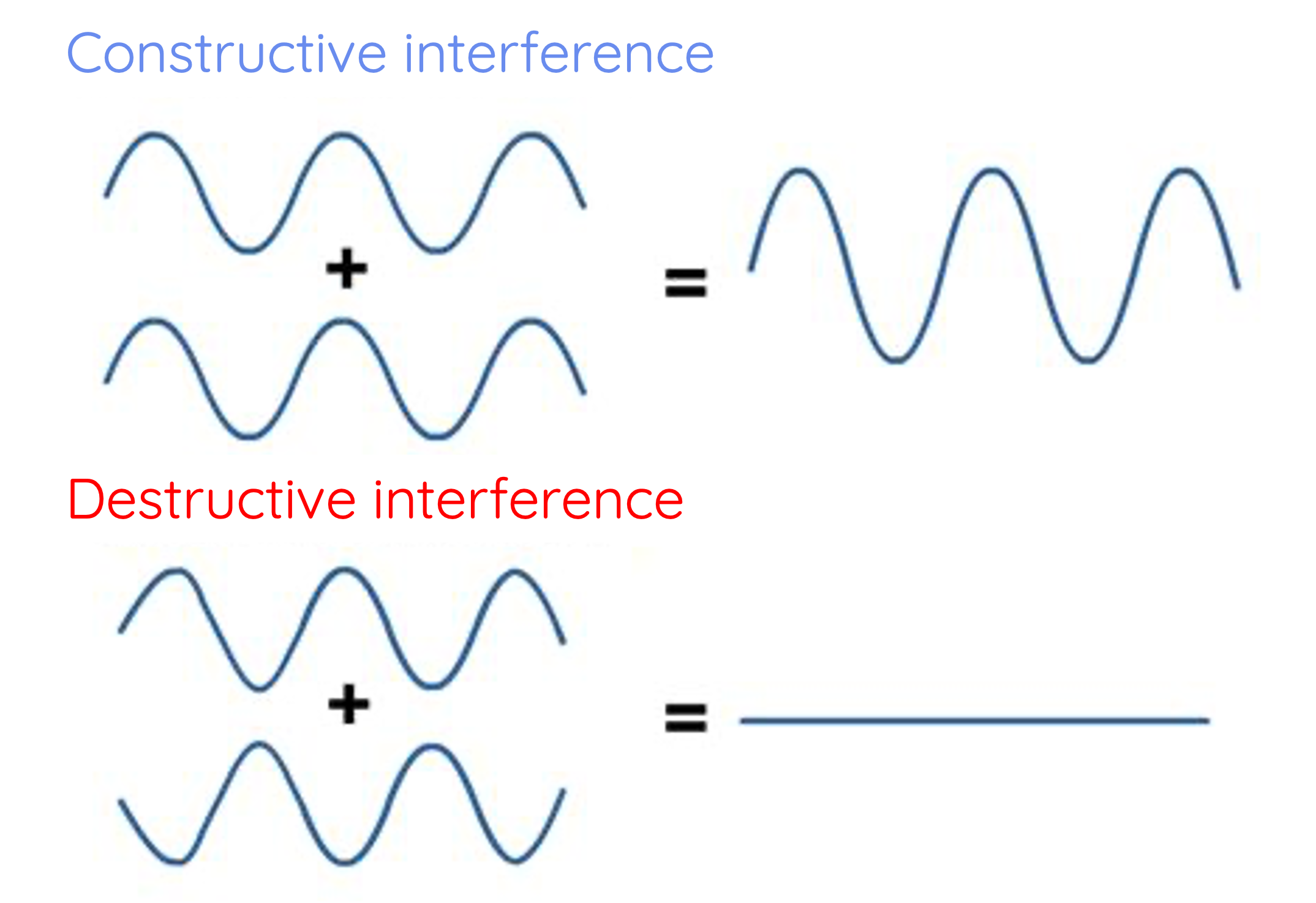# Wave Behaviour: Reflection, Refraction, Diffraction and Superposition

This topic is part of the HSC Physics course under the section Wave Behaviour.

### HSC Physics Syllabus

• explain the behaviour of waves in a variety of situations by investigating the phenomena of:

– reflection
– refraction
– diffraction
– wave superposition (ACSPH071, ACSPH072)

### Understanding Wave Behaviour

Wave behaviour is a crucial aspect of Year 11 HSC Physics. It encompasses several phenomena, including reflection, refraction, diffraction, and wave superposition. Understanding these principles can give us insights into everything from the way light interacts with materials to the propagation of sound waves.

### Reflection

Reflection is the phenomenon where waves bounce off a surface, reversing their direction. This behaviour is common in light and sound waves and can be observed in everyday life.

Law of Reflection

The angle of incidence (the angle between the incoming wave and the normal of the surface) is equal to the angle of reflection (the angle between the reflected wave and the normal). The normal is a perpendicular line drawn from the surface.The law of reflection is stated as:

$$\theta_{i} = \theta_{r}$$

There are two main types of reflection:

• Specular Reflection: This occurs on smooth surfaces, like mirrors, where waves are reflected uniformly.
• Diffuse Reflection: This takes place on rough surfaces, causing waves to scatter in different directions.

### Refraction

Refraction is the bending of waves when they pass through different media, altering their speed and direction.

Snell's Law

Snell's Law provides a mathematical way to predict the angle of refraction based on the medium's refractive index. It's expressed as:

$$n_1\sin{\theta_1} = n_2 \sin{\theta_2}$$

Where n_1 and n_2 are the refractive indices of the two media, and \theta_1 and \theta_2 are the angles of incidence and refraction, respectively.If the refractive index of the second medium is greater than the first medium, then the angle of refraction would be smaller than the angle of incidence. This means the wave would bend towards the normal as it travels through the medium.

Vice versa, if the refractive index of the second medium is smaller than the first medium, the wave would bend away from the normal as it travels through the medium.

### Refraction: Effect on Speed, Frequency, and Wavelength

Refraction changes the speed of a wave as it passes from one medium into another, but it does so without altering the wave's frequency.

The speed of a wave is given by:

$$v = f \times \lambda$$

When a wave passes from one medium to another with a different refractive index, its speed changes. This change in speed leads to refraction, or the bending of the wave. But what happens to the frequency and wavelength of the wave during this process?

#### Frequency Remains Constant

The frequency of a wave is determined by the source of the wave and remains constant as the wave passes through different media. Since the frequency doesn't change, any alteration in speed must be compensated for by a corresponding change in wavelength.

#### Wavelength Changes

The wavelength of the wave changes in proportion to the speed of the wave as it enters a new medium. If the wave slows down (as it does when moving from a medium with a lower refractive index to one with a higher refractive index), the wavelength decreases. Conversely, if the wave speeds up (when moving from a higher to a lower refractive index), the wavelength increases.

### Diffraction

Diffraction refers to the spreading out of waves as they pass through an opening or around obstacles.When waves pass through a narrow slit, they spread out in a pattern that can be described using the wavelength and the size of the opening. As the size of the opening becomes smaller, the degree of diffraction increases.

More detail on diffraction and double slit diffraction are discussed separately here.

### Wave Superposition

Wave superposition is the phenomenon where two or more waves combine to form a resultant wave. The resultant wave's amplitude at any point is the sum of the amplitudes of the individual waves at that point.Constructive interference occurs when waves meet in phase, their amplitudes add together, creating a wave with a larger amplitude.

Destructive interference occurs when waves meet out of phase, their amplitudes cancel out, creating a wave with a smaller or even zero amplitude.